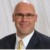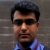# What You May Not Know About Adding Variances

ByJustin D. Fisk

Imagine for a moment that your Six Sigma project does not have the rigorous measurement system you would like it to have, and you have to calculate your primary metric from a few inputs instead of measuring it directly. Or perhaps you are designing a new product and want to understand how the variability in your raw materials will drive variability in the finished product.

To start addressing either of those issues, anyone who has completed Six Sigma training probably has a basic idea of how to combine input variances and arrive at the variance in the output. Black Belts are taught that you add the variances. Then you take the square root of the resulting variance to compute the standard deviation. Six Sigma instructors drill this idea into their students telling them time and time again that we add variances not standard deviations.

### Relying on the Linearity of the Equation

What you may not have learned is that this additive property relies on the linearity of the equation relating the distributions together. If the equation is quadratic, or some other non-linear combination, or if the coefficients of the transfer function are not equal to one, there is more to combining the variances than just summing them. This discussion of the equations behind combining variances looks at three examples – two where the transfer function is linear and one where it is not.

To discuss the equations governing the addition of variances, one must assume that the transfer function for a process can be obtained through statistically designed experiments or other means. Now consider the basic equation in all Six Sigma projects:

y = f ( x1, x2, x3,…xn ) (Equation 1)

Equation 1 states that the output, y, is a function of various inputs, or x values. In order to calculate the variance in the output based on the variance in all of the inputs, the equation is as follows:

sy2 = S [(d f/ xi )2 * sxi2] summed for i = 1 to i = n (Equation 2)

This equation is the part you may not have seen before. In words, it states that the total variance in the output is equal to the sum of the contributions from all of the inputs. It further states that the contribution of each input is equal to the partial derivative of the function with respect to that input, which is then multiplied by the standard deviation of that input, and then that quantity is squared to put it in terms of variance. (Note that Equation 2 uses the first order terms of a Taylor Series expansion, and assumes that all inputs are normally, or at least symmetrically, distributed. Equation 2 also assumes that the inputs are not correlated, meaning that there is no covariance among them.) Now Equation 2 will be applied to three different examples.

### Example 1

Consider the case of a linear transfer function with all coefficients equal to one as shown in Equation 3.

y = x1 + x2 (Equation 3)

In this example, because the partial derivatives with respect to each of the inputs are equal to one, the variances of the input values can be added to arrive at the variance in the output. Stated mathematically:

Handpicked Content:   What Is a Stable Process?

sy2 = sx12 + sx22 (Equation 4)

This case, where the variances in the inputs are simply added together to obtain the variance in the output, is the most basic example. However, be aware that combining variances this way requires that the transfer function is linear and that the coefficients of that transfer function all equal one.

### Example 2

Now consider the case of a linear transfer function with coefficients not equal to one, such as Equation 5.

y = 3x1 + 4x2 (Equation 5)

In this case, the partial derivative of the output with respect to the first input, x1, is equal to 3, while the partial derivative of the output with respect to the second input, x2, is 4. Applying Equation 2:

sy2 = (3)2 * sx12 + (4)2 * sx22 (Equation 6)

or, simplifying:

sy2 = 9sx12 + 16sx22 (Equation 7)

In this case, the value of the coefficients in the transfer function affect the coefficients in the variance formula.

### Example 3

Finally, consider a non-linear transfer function. While this could include trigonometric functions, this example focuses on the transfer function given in Equation 8.

y = 5x12 + 4x23 + 8x1x2 (Equation 8)

Notice here that the third term, 8x1x2, most likely arises from a statistically significant interaction between the two inputs. Because the partial derivatives in this example are not trivial, they are shown in Equations 9 and 10.

dy /dx1 = 10x1 + 8x2 (Equation 9)

dy / dx2 = 12x22 + 8x1 (Equation 10)

Thus, applying Equation 2 yields the relationship between the variances in the inputs and the variance in the output, as shown in Equation 11.

sy2 = (10x1 + 8x2)2 * sx12 + (12x22 + 8x1)2 * sx22 (Equation 11)

This case is interesting because the values of the inputs, x1 and x2, must be known in order to determine the relationship between the input and output variances. Because the previous two examples were linear, the relationship between input and output variances did not change, regardless of the values of the inputs. (Also, it is important to note that, in practice the input values to be plugged into the formula for output variance are the means of the inputs themselves.)

So with values for the inputs given in Equations 12 and 13, we can substitute into Equation 11 and determine the formula relating our input and output variances, Equation 14. Equation 15 shows the final relationship after simplification.

Assume x1 = 4 (Equation 12)

Assume x2 = 3 (Equation 13)

sy2 = (40 + 24)2 * sx12 + (108 + 32)2 * sx22 (Equation 14)

sy2 = 4096 * sx12 + 19600 * sx22 (Equation 15)

This example could be taken a step further and provide values for the variance in the two inputs. However, to keep it simple, let us assume that the variances in the inputs are equal. Then we would know that x2 drives 82.7 percent of the output variation, which is calculated by dividing 19600 by (4096 + 19600).

The key lesson in this example is that for a non-linear transfer function the mean values of the inputs are needed in order to calculate the variance in the output. In addition, the partial derivatives with respect to the various inputs can become much more involved with trigonometric or higher order functions.

Handpicked Content:   Estimating Sample Size for Process Capability with Special Causes (with Template)

This article has attempted to: First, reinforce the importance of understanding the underlying theory and assumptions before applying any Six Sigma concept; and second, emphasize the importance of understanding the underlying transfer function and input values before adding variances. As a Six Sigma practitioner, hopefully you will think twice next time you add variances, considering linearity and the coefficients in the transfer function, so that you do not make a mistake that could significantly affect the validity and impact of your Six Sigma project.

##### Justin D. Fisk

1.Liver enzymes

As a Six Sigma practitioner, hopefully you will think twice next time you add variances, considering linearity and the coefficients in the transfer function, so that you do not make a mistake that could significantly affect the validity and impact of your Six Sigma project.

2.Paul Winstone

Thanks for the refresher.

3.Rene Klerx

Propagation of error is a base for sensitivity analysis. This variation is sensitive to initial settings. An important part in the DfSS curriculum and robust deesign.

4.Amit Kumar Ojha

A very insightful article Justin. It reminds that six sigma is a disciplined and structured approach which is based on sound mathematical theories. Also since in real life scenarios most of the transfer function are not linear, the thrird example will be immensely useful.

5.Joel

Good article and knowledge for belts to keep in mind. I’d also add that the additive variances assume that the X’s are independent which often is not the case.

6.Carl Berardinelli

Great article, thanks. Most Belt students would “check out” if you started talking about partial derivatives. How do you ovecome this when you teach classes?